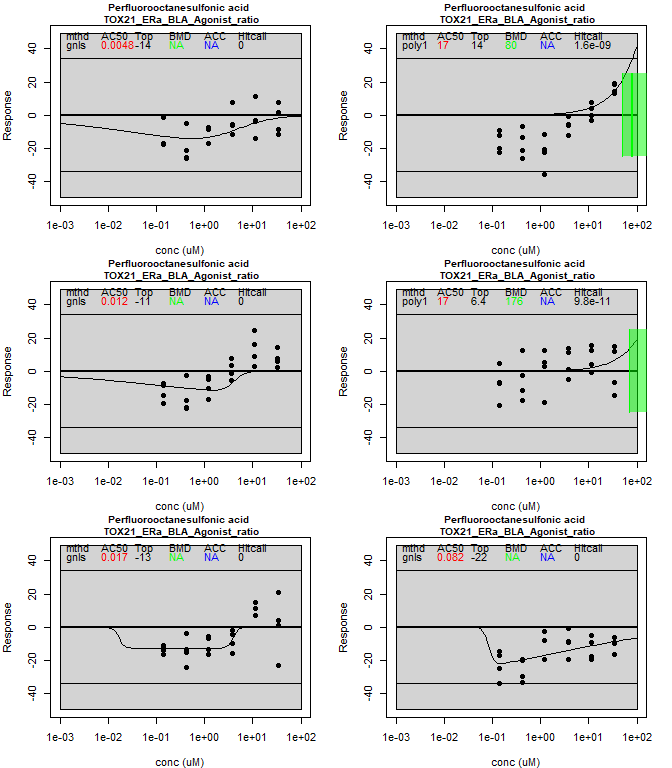# Getting started with tcplfit2

The package tcplfit2 contains the core concentration-response functionality of the package tcpl (The ToxCast Pipeline) built to process all of the ToxCast high-throughput screen (HTS) data at the US EPA. Much of the rest of the code in tcpl is used to do data processing, normalization, and database storage. We wanted to reuse the core concentration-response code for other projects, and add extensions to it, which was the origin of the current package tcplfit2. The main set of extensions was to include all of the concentration-response models that are contained in the program BMDExpress. These include exponential, polynomial (1 & 2), and power functions in addition to the original Hill, gain-loss and constant models. Additionally, we wanted to include BMD (Benchmark Dose Modeling) outputs, which is simply defining a Benchmark Response (BMR) level and setting the BMD to the concentration where the curve crosses the BMR level. One final addition was to let the hitcall value be a continuous number ranging from 0 to 1. Continuous hitcall in tcplfit2 are defined as the product of three proportional weights: 1) the AIC of the winning model is better than the constant model (i.e. winning model is not fit to background noise), 2) at least one concentration has a median response that exceeds cutoff, and 3) the top from the winning model exceeds the cutoff. This vignette describes some functionality of the tcplfit2 package with a few simple examples.

## Example 1: Running a single concentration-response calculation

All calculations use the function concRespCore which has several key inputs. The first set are put into a named list called ‘row’:

• conc - a vector of concentrations (not log concentrations)
• resp - a vector of responses, of the same length as conc. Note that replicates are allowed, i.e. there can be multiple pairs of conc and resp with the same concentration value.
• cutoff- this is the value that the response must exceed before a a curve can be called a hit. For ToxCast, this is usually some multiple (typically 3) of the median absolute deviation (BMAD) around baseline for the lowest two concentration. The user is free to make other choices
• bmed - this is the median of the baseline. The entire response series will be shifted by this amount. Set to zero if the data is zero-centered.
• onesd- This is one standard deviation of the noise around the baseline. The BMR value = onesd*bmr_scale. The default bmr_scale is 1.349.

The function concRespCore can also have other optional elements which will be included in the output. These can be, for instance, the name of the chemical (or other identifiers) or the name of the assay being modeled. Two other parameters might be used. The first is a Boolean conthits. If TRUE (the default, and recommended usage), the hitcall returned will be a continuous value between 0 and 1. The other is do.plot. If this is set to TRUE (default is FALSE), a plot of the curve will be generated. The user can also select only a subset of the models to be run. The example below has all of the possible ones included. If the fitmodels parameter is specified, then it must always include the constant model (cnst) since this provides one basis for comparison during hitcalling. However, some models may be excluded, for example the gain-loss (gnls) model is excluded in some applications.

To run a simple example, use the following code …

  conc <- list(.03,.1,.3,1,3,10,30,100)
resp <- list(0,.2,.1,.4,.7,.9,.6, 1.2)
row = list(conc = conc, resp = resp, bmed = 0, cutoff = 1, onesd = .5,name="some chemical")
on.exit(par(oldpar))
par(xpd = TRUE)
res <- concRespCore(row,fitmodels = c("cnst", "hill", "gnls", "poly1", "poly2", "pow", "exp2", "exp3",
"exp4", "exp5"),conthits = T, do.plot=T)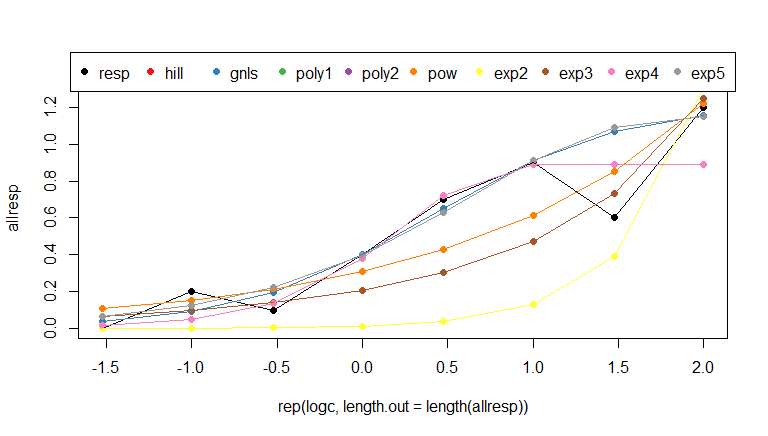The output of this run will be a data frame with one row, summarizing the results for the winning model.

## Example 2: Running a series of concentration-response models for a single assay

The input data for this example is taken from one of the Tox21 HTS assays, for estrogen receptor (ER) agonist activity. The data is from the mc3 table in the database invitrodb, which is the back end for tcpl. The input data for this example have already been through the pre-processing steps (prior to tcpl), and processed through several steps in the ToxCast pipeline (including normalization of concentration data and transformation of response values - Levels 0 - 3). This example will run 6 chemicals out of the 100 that are included in the data set, and will create plots for these. The plotting routine concRespPlot is somewhat generic, and we anticipate that users will make their own version of this. To run this example, use the following code …

  # read in the data
data("mc3")

# set up a 3 x 2 grid for the plots
on.exit(par(oldpar))
par(mfrow=c(3,2),mar=c(4,4,2,2))

# determine the background variation
temp <- mc3[mc3$logc<= -2,"resp"] bmad <- mad(temp) onesd <- sd(temp) cutoff <- 3*bmad # select six samples. Note that there may be more than one sample processed for a given chemical spid.list <- unique(mc3$spid)
spid.list <- spid.list[1:6]

for(spid in spid.list) {
# select the data for just this sample
temp <- mc3[is.element(mc3$spid,spid),] # The data file has stored concentration in log10 form, so fix that conc <- 10**temp$logc
resp <- temp$resp # pull out all of the chemical identifiers and the name of the assay dtxsid <- temp[1,"dtxsid"] casrn <- temp[1,"casrn"] name <- temp[1,"name"] assay <- temp[1,"assay"] # create the row object row <- list(conc = conc, resp = resp, bmed = 0, cutoff = cutoff, onesd = onesd,assay=assay,dtxsid=dtxsid,casrn=casrn,name=name) # run the concentration-response modeling for a single sample res <- concRespCore(row,fitmodels = c("cnst", "hill", "gnls", "poly1", "poly2", "pow", "exp2", "exp3", "exp4", "exp5"),conthits = T, aicc = F,bidirectional=F) # plot the results concRespPlot(res,ymin=-10,ymax=100) }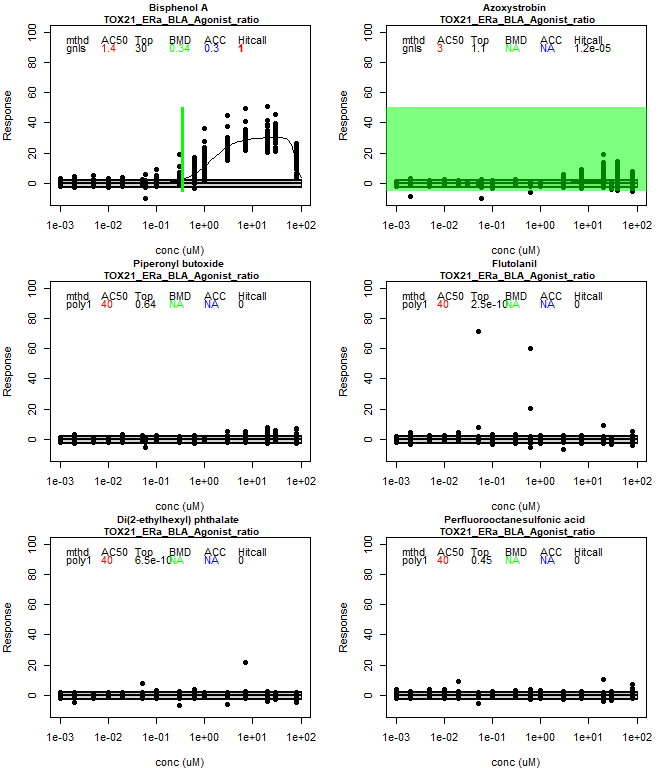One would typically save the result rows in a data frame end export these for further analysis. You could remove the plotting function from the current loop and have a loop that read from the overall results data frame and only plot selected results (e.g. those with significant responses). ## Example 3: Plotting concentration-response modeling on transcriptional signatures The input data for this example contains 6 signatures for one chemical in a transcriptomics data set. Each signature is a different assay endpoint, thus one row in the data represents a given chemical and signature pair (assay endpoint). This data set is a sample from the signature scoring method that provides the cutoff, one standard deviation, and the concentration-response data. The example illustrates two kinds of plots available in tcplfit2. In the call to concRespCore(), the argument do.plot is set to TRUE, which provides a simple plot showing results of all the different curve fitting methods. Next, utilizing the function concRespPlot() provides a more informative plot for the winning model.  # call additional R packages library(stringr) # string management package # read in the file data("signatures") # set up a 3 x 2 grid for the plots oldpar <- par(no.readonly = TRUE) on.exit(par(oldpar)) par(mfrow=c(3,2),mar=c(4,4,2,2)) # fit 6 observations in signatures for(i in 1:nrow(signatures)){ # set up input data row = list(conc=as.numeric(str_split(signatures[i,"conc"],"\\|")[]), resp=as.numeric(str_split(signatures[i,"resp"],"\\|")[]), bmed=0, cutoff=signatures[i,"cutoff"], onesd=signatures[i,"onesd"], name=signatures[i,"name"], assay=signatures[i,"signature"]) # run concentration-response modeling (1st plotting option) out = concRespCore(row,conthits=F,do.plot=T) if(i==1){ res <- out }else{ res <- rbind.data.frame(res,out) } }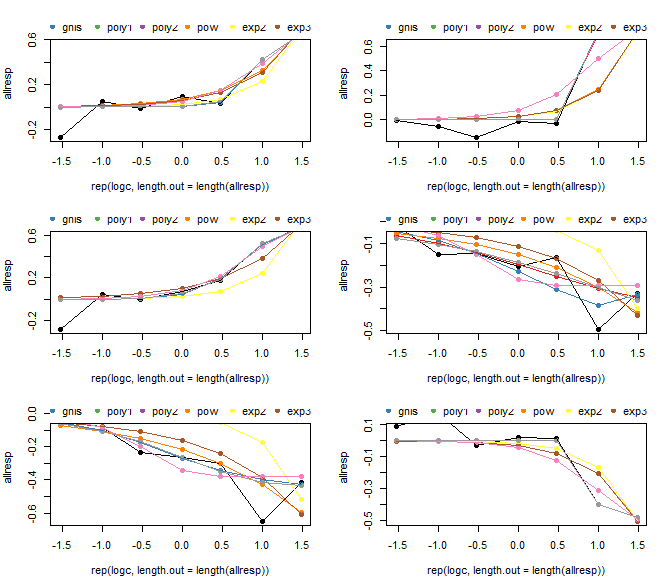# set up a 3 x 2 grid for the plots oldpar <- par(no.readonly = TRUE) on.exit(par(oldpar)) par(mfrow=c(3,2),mar=c(4,4,2,2)) # plot results using concRespPlot(2nd plotting option) for(i in 1:nrow(res)){ concRespPlot(res[i,],ymin=-1,ymax=1) }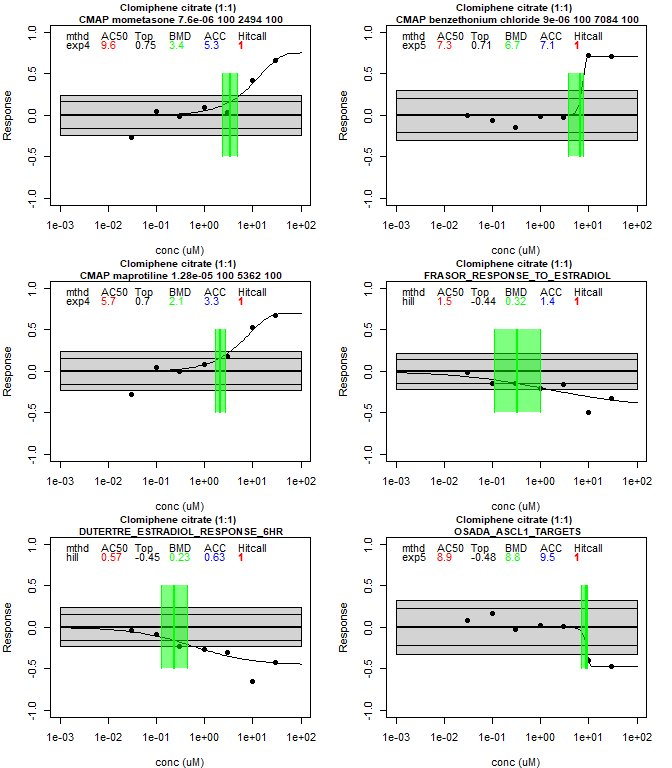## Example 4: Running tcpl-like multi-concentration response data without a database connection The ToxCast pipeline tcpl is an R package that manages, curve-fits, plots, and stores ToxCast data to populate its linked MySQL database, InvitroDB. The original tcplFit() function within tcpl performed basic concentration response curve fitting. Processing with tcpl_v3 and beyond depends on tcplfit2 to allow a wider variety of concentration-response models when using invitrodb in the 4.0 schema and beyond. tcplLite was deprecated with the updates to tcpl and development of tcplfit2, since tcplfit2 allows one to perform curve-fitting and hit-calling independent of a database. The example below demonstrates how to perform an analogous tcplLite analysis with tcplfit2. For additional information, please consult vignettes for library(tcpl) at https://CRAN.R-project.org/package=tcpl. The input for this example comes from the ACEA_AR assay. Data from the assay component ACEA_AR_agonist_80hr was analyzed in the positive analysis fitting direction relative to DMSO as the neutral control and baseline of activity. Using a electrical impedance as a cell growth reporter, increased activity can be used to infer increased signaling at the pathway-level for the androgen receptor (as encoded by the AR gene). Given heterogeneous assay data, source data often must go through pre-processing steps to transform into a uniform data format, often like this level 0. The below table is identical to the multi-concentration level 0 data (mc0) table that would be seen in invitrodb and recognized by tcpl. Columns include: • m0id = Level 0 id • spid = Sample id • acid = Unique assay component id; unique numeric id for each assay component • apid = Assay plate id • coli = Column index (location on assay plate) • rowi = Row index (location on assay plate) • wllt = well type • wllq = well quality • conc = concentration • rval = raw value • srcf = Source file name • clowder_uid = clowder unique id for source files • git_hash = hash key for pre-processing scripts # Loading in the level 0 example data set from invitrodb data("mc0") library(data.table) dat <- mc0 DT::datatable(head(dat[wllt=='t',]),rownames= FALSE, options = list(scrollX = T)) To run standalone tcplfit2 fitting without the need for a MySQL database connection like invitrodb, the user will replicate stepping through the multiple levels of processing. A detailed explanation of processing levels can be found within tcpl’s Data Processing vignette. Level 1 importantly establishes the concentration index. The concentration index is simply the distinct concentrations ranked from lowest to highest, and this index can be used to calculate the baseline median absolute deviation for an assay. library(tcpl) #> Warning: package 'tcpl' was built under R version 4.2.3 #> tcpl (v3.1.0) loaded with the following settings: #> TCPL_DB: C:/Users/jbrown20/R-4.2.2/library/tcpl/csv #> TCPL_USER: NA #> TCPL_HOST: NA #> TCPL_DRVR: tcplLite #> Default settings stored in tcpl config file. See ?tcplConf for more information. ## Order by the following columns setkeyv(dat, c('acid', 'srcf', 'apid', 'coli', 'rowi', 'spid', 'conc')) ## Define replicate id (rpid) column for test compound wells nconc <- dat[wllt == "t" , ## denotes test well as the well type (wllt) list(n = lu(conc)), #total number of unique concentrations by = list(acid, apid, spid)][ , list(nconc = min(n)), by = acid] dat[wllt == "t" & acid %in% nconc[nconc > 1, acid], rpid := paste(acid, spid, wllt, srcf, apid, "rep1", conc, sep = "_")] dat[wllt == "t" & acid %in% nconc[nconc == 1, acid], rpid := paste(acid, spid, wllt, srcf, "rep1", conc, sep = "_")] ## Define rpid column for non-test compound wells dat[wllt != "t", rpid := paste(acid, spid, wllt, srcf, apid, "rep1", conc, sep = "_")] ## set repid based on rowid dat[, dat_rpid := rowid(rpid)] dat[, rpid := sub("_rep[0-9]+.*", "",rpid, useBytes = TRUE)] dat[, rpid := paste0(rpid,"_rep",dat_rpid)] # Define concentration index indexfunc <- function(x) as.integer(rank(unique(x))[match(x, unique(x))]) dat[ , cndx := indexfunc(conc), by = list(rpid)] ### Adjustments Levels 2 and 3 are used for data adjustments and normalization. Generally if the response values (rval) need to be logged or transformed in some way from their original values this is where that adjustment would occur. Transformed response values are referred to as corrected values and are stored in the cval field/variable. However, in this case, the corrected values (cval) are identical to the original response values (rval). # If no adjustments are required for the data, the corrected value (cval) should be set as original rval dat[,cval := rval] ## Poor well quality (wllq) wells should be removed dat <- dat[!wllq == 0,] ## Fitting generally cannot occur if response values are NA therefore values need to be removed dat <- dat[!is.na(cval),] ## A column for log10 concentration is added as some of the mc3 methods require logc. Given logging concentration, conc=0 are not allowed therefore a dummy non-zero value should be used dat[conc == 0 , conc := 0.0001] dat[ , logc := log10(conc)] #As a final step to prepare the dataset tcplfit2 processing, a dummy aeid is required if using mc3_mthds from tcpl dummy_aeid <- 99999 dat[,aeid := dummy_aeid] ## Set aeid as a key setkey(dat,aeid) Once the data is initialized to a point where the required fields are available, the methods included in the tcpl package can be identified and applied without the need for a database connection. You can see the list of available methods for Level 3 in the table below: mthd_funcs <- tcpl:::mc3_mthds() DT::datatable(tcpl::tcplMthdList(3),rownames= FALSE, options = list(scrollX = T)) ### Normalization Here three normalization methods are selected and applied to the data. Note because of the way tcpl handles the application of functions, the dataframe must be called dat. In the future, tcpl will export these functions so that they can be applied to any dataset without the need for a specific name or dummy aeid. # apply level 3 methods ## These methods directly apply the normalization methods from tcpl without the need for a DB connection lapply(mthd_funcs[["bval.apid.nwlls.med"]](dummy_aeid), eval) lapply(mthd_funcs[["pval.apid.medncbyconc.min"]](dummy_aeid),eval) lapply(mthd_funcs[["resp.pc"]](dummy_aeid),eval) Level 4 determines the baseline variability, or noise, that will later be used for cutoff calculation. Using the established concentration index, the level 4 methods can be loaded in a similar way to level 3. mthd_funcs_l4 <- tcpl:::mc4_mthds() DT::datatable(tcpl::tcplMthdList(4), rownames= FALSE, options = list(scrollX = T)) There are much fewer level 4 methods, but generally it is a requirement to assign a method that calculates the bmad and assign a method that calculates the standard deviation of the noise for tcplfit2 fitting. # apply level 4 methods ## These methods directly apply the noise calculation and fitting methods from tcpl without the need for a DB connection lapply(mthd_funcs_l4[["bmad.aeid.lowconc.twells"]](),eval) lapply(mthd_funcs_l4[["onesd.aeid.lowconc.twells"]](),eval) lapply(mthd_funcs_l4[["bidirectional.false"]](),eval) ### Dose-Response Curve Fitting After methods up to level 4 have been applied, the model fitting can begin. In tcpl, this would be considered level 4, and is where tcplfit2 is used to fit all of the models as a dependency for tcpl. #do tcplfit2 fitting myfun <- function(y) { res <- tcplfit2::tcplfit2_core(y$conc,
y$resp, cutoff = unique(y$bmad),
bidirectional = TRUE,
verbose = FALSE,
force.fit = TRUE,
fitmodels = c("cnst", "hill", "gnls", "poly1",
"poly2", "pow", "exp2", "exp3",
"exp4", "exp5")
)
list(list(res)) #use list twice because data.table uses list(.) to look for values to assign to columns
}

The following code performs dose-response modeling for all spids in the dataset. Warning: The fitting step for the full data set, dat, can take 7-10 minutes to run. Hence the code chunk following provides a subset example of data for curve fitting and hitcalling. The subset data only contains records of six samples.

# only want to run tcplfit2 for test wells in this case
# this chunk doesn't run, fit the curves on the subset below
dat[wllt == 't',params:= myfun(.SD), by = .(spid)]
# create a subset that contains 6 samples and run curve fitting
subdat <- dat[spid %in% unique(spid)[10:15],]
subdat[wllt == 't',params:= myfun(.SD), by = .(spid)]

### Continuous Hitcalling

After all of the models have been fit, hitcalling can occur. The output of level 4 can be fed directly into the tcplhit2_core function. The results are then pivoted and shown in the resulting datatable.

myfun2 <- function(y) {
res <- tcplfit2::tcplhit2_core(params = y$params[], conc = y$conc,
resp = y$resp, cutoff = 3*unique(y$bmad),
onesd = unique(y$osd) ) list(list(res)) } # continute with hitcalling res <- subdat[wllt == 't', myfun2(.SD), by = .(spid)] #pivot wider res_wide <- rbindlist(Map(cbind, spid = res$spid, res\$V1))

DT::datatable(res_wide,options = list(scrollX = T))

Hitcalling can also be done with the full data set, dat.

The output table resulting from the previous code chunk is the same format as the res table in example 3. Thus, one can use the concRespPlot function, as done previously in example 3, to plot the results. The next code chunk demonstrates how to visualize the example 4 fit results.

  # set up a 3 x 2 grid for the plots
# plot results using concRespPlot(2nd plotting option)
}